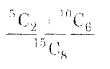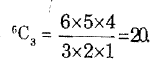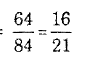# Engineering Maths - Probability

>>>>>>>>Probability

• A

140/429• B

129/440• C

139/420• D

none of these• Option : A
• Explanation : Number of ways in which 8 balls can be drawn
out of 15 is 15C8
Number of ways of drawing 2 red balls is 5C2
and corresponding to each of these 5C2 ways
of drawing a red ball, there are IOC5 ways of
drawing 6 black balls.
Hence total number of ways in which 2 red
and 6 black balls can be drawn = 5C2 X 10C6

Required Probability ==  140/429

• A

7: 16• B

16: 7• C

6: 14• D

14: 6• Option : A
• Explanation : A can draw a ticket in 3C1 = 3 ways.
Number of cases in which A can get a prize
is clearly 1.

Probability of A's success = 1/3

Again B can draw a ticket inNumber of ways in which B gets all blanksNumber of ways of getting a prize
= 84 - 20 = 64

Thus, probability of B's success  =A's probability of success : B's probability
of success• A

independent• B

dependent• C

both (a) and (b)• D

none of these• Option : A
• Explanation : P(A ∩ B') =  P(A) - P(A ∩ B)

= P(A) - P(A) P(B)

= P(A) (1 - P(B)) = P(A) P(B')

Hence, A and B' are independent.

• A

7/2• B

2/7• C

3/7• D

7/2• Option : B
• Explanation : A leap year consists of 366 days, so that there
are 52 full weeks (and hence 52 Sundays) and
two extra days. These two days can be
(i) Monday, Tuesday
(ii) Tuesday, Wednesday,
(iii) Wednesday, Thursday
(iv) Thursday, Friday
(v) Friday, Saturday
(vi) Saturday, Sunday
(vii) Sunday, Monday
Of these 7 cases. the last two are favourable

Required probability = 2/7

• A

True• B

False• Option : A
• Explanation :Related Quiz.
Probability